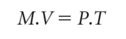# identity

(redirected from identity element)
Also found in: Dictionary, Thesaurus, Medical, Legal, Encyclopedia, Wikipedia.
Related to identity element: Inverse element

## identity

a means of portraying arithmetically the enduring equality between two (or more) VARIABLES that are equal by definition. For example, £1 = 100p (or \$1 = 100¢), and no matter how many pounds (or dollars) we have, they can always be converted into pennies (or cents) by multiplying by 100. Identities are generally given a three-bar ‘identity’ sign (=) to indicate that the value to the left of the three bars is identical to the value to the right of the sign. The QUANTITY THEORY OF MONEY is one of the best-known examples of an identity in economics, written as:where M is the money stock, V is the velocity of circulation of money, P is the general price level and T is the number of transactions undertaken. See EQUATION.

Collins Dictionary of Economics, 4th ed. © C. Pass, B. Lowes, L. Davies 2005
References in periodicals archive ?
Then (RNQ(H), [symmetry]) is a hypergroup with identity element [bar.0] = (0, [p.summation over (i=1)] 0[T.sub.i], [R.summation over(j=1)] 0[I.sub.j], [r.summation over(k=1)] 0[F.sub.k]) if and only if (H, +) is a hypergroup with identity element 0.
and the connected component of the identity element equals the whole group.
To get a meaningful definition, we cannot let Magnus choose the identity element. The token starts at the identity element of G.
Similarly, n-ary semigroup (R, g) has an identity element 1 if
It is also easy to see that s * t [member of] H is the identity element of ([G.sub.H], [omicron]).
However our understanding is that monoids include an identity element and semigroups do not.
Since [a.sup.2][[omicron].sub.x] = ([R.sup.-1.sub.a][a.sup.2])([L.sup.-1.sub.a]x) = a([L.sup.-1.sub.a]x) = [L.sub.a][L.sup.-1.sub.a]x = x and similarly x [omicron] [a.sup.2] = x for all x [member of] B, [a.sup.2] is an identity element in B.
It follows that A is the identity element of this structure by symmetry.
Let U be the complexified universal enveloping algebra of the real Lie algebra g of G; which is canonically isomorphic onto the algebra of all distributions on G supported by {0} , where 0 is the identity element of G.
In some analyses, a salient identity element has been MHC's placement on the occupation-profession continuum (cf.
Part 2 contains chapters that address identity in earl y, middle, and late adolescence, as well as a further chapter of selected issues posing additional identity challenges for a substantial number of adolescents in the general population; all of these special challenges involve coming to terms with difference resulting from a nonchosen identity element. Part 3 contains chapters addressing identity development during the early, middle, and later years of adulthood.

Site: Follow: Share:
Open / Close